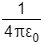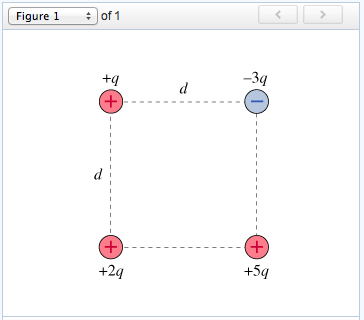# Problem: Four point charges form a square with sides of length d, as shown in the figure. In the questions that follow, use the constant k in place of .What would be the kinetic energy K2q of charge 2q at a very large distance from the other changes?Express your answer in terms of q, d, and appropriate constants.

###### FREE Expert Solution

Electric potential energy:

$\overline{){\mathbf{U}}{\mathbf{=}}\frac{\mathbf{k}{\mathbf{q}}_{\mathbf{1}}{\mathbf{q}}_{\mathbf{2}}}{\mathbf{r}}}$

The charge 2q is initially at rest while in the system. At a large distance, the potential energy of charge 2q as part of the system is zero. From the conservation of energy:

U0 + KE0 = Uf + KEf

The potential energy of charge 2q in the system = U0

Uf = 0

KE0 = 0###### Problem Details

Four point charges form a square with sides of length d, as shown in the figure. In the questions that follow, use the constant k in place of.What would be the kinetic energy K2q of charge 2q at a very large distance from the other changes?